Get Started »

# Mathematical Typesetting

Use keyboard shortcuts (such as CTRL+/ for fractions) to insert fillable typeset expressions:

(Click a box to highlight and fill it or press TAB to move among the boxes.)This is a simple way to enter exponents (CTRL+6), subscripts (CTRL+-) and other common expressions.

Pressing the ESC key in a notebook generates the `` symbol for keyboard shortcuts of the form ``alias`` (often notated ESCaliasESC in documentation).

Type the correct alias, and the expression changes when the closing `` is entered:

(The alias for “partial derivative” is “pd”.)Sums, integrals and other high-level expressions are generated this way:

(The alias for “definite integral” is “dintt”.)Many Greek letters and other special characters also use this form.

From a desktop notebook, you can select the “Basic Math Assistant” from the Palettes Menu for an overview of available typeset forms.

Clicking a palette button inserts a fillable expression at your cursor’s position: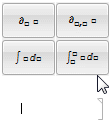Use the TraditionalForm command to display any expression in traditional mathematical notation:

 In:=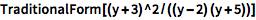⨯ `TraditionalForm[(y + 3)^2/((y - 2) (y + 5))]`
 Out=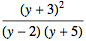Convert existing cells to TraditionalForm using SHIFT+CMD+T:

(Expressions in TraditionalForm are still computable.)
 In:=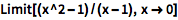⨯ `Limit[(x^2 - 1)/(x - 1), x -> 0]`
 In:=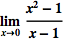⨯ ```\!\(\*UnderscriptBox[\(lim\), \(x \[Rule] 0\)]\)( x^2 - 1)/(x - 1)```
 Out=QUICK REFERENCE: Mathematical Typesetting `»`